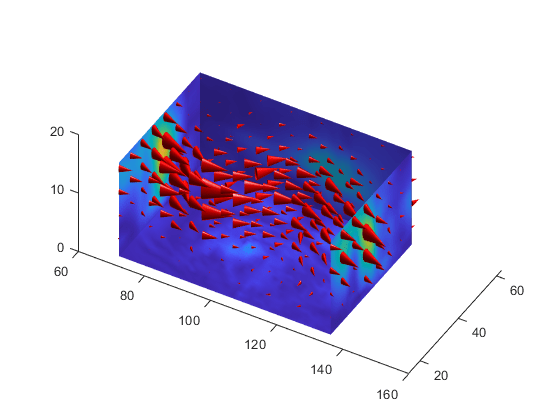# coneplot

•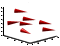## 语法

```coneplot(X,Y,Z,U,V,W,Cx,Cy,Cz) coneplot(U,V,W,Cx,Cy,Cz) coneplot(...,s) coneplot(...,color) coneplot(...,'quiver') coneplot(...,'method') coneplot(X,Y,Z,U,V,W,'nointerp') coneplot(axes_handle,...) h = coneplot(...) ```

## 说明

`coneplot(X,Y,Z,U,V,W,Cx,Cy,Cz)` 以指向速度向量方向的圆锥体形式绘制速度向量，并且具有与速度向量的量级成比例的长度。 `X``Y``Z` 定义向量场的坐标。`U``V``W` 定义向量场。这些数组必须为大小相同的单调数组，并表示轴对齐的笛卡尔网格（例如 `meshgrid` 生成的数据）。`Cx``Cy``Cz` 定义向量场中圆锥体的位置。可视化方法中的指定流线图的起点部分提供了有关定义起始点的详细信息。

`coneplot(U,V,W,Cx,Cy,Cz)`（省略 `X``Y``Z` 参数）假定 `[X,Y,Z] = meshgrid(1:n,1:m,1:p)`，其中 `[m,n,p]= size(U)`

`coneplot(...,s)` 将自动缩放圆锥体以适应图形，然后按缩放因子 `s` 对圆锥体进行拉伸。如果未为 `s` 指定值，则 `coneplot` 使用值 1。使用 `s = 0` 绘制圆锥体，无需自动缩放。

`coneplot(...,color)` 在向量场上对数组 `color` 插值，然后根据插入的值对圆锥体进行着色。`color` 数组的大小必须与 `U``V``W` 数组的大小相同。此选项仅对圆锥体有效（即不能与 `quiver` 选项一起使用）。

`coneplot(...,'quiver')` 绘制箭头而不是圆锥体（有关箭头图的说明，请参阅 `quiver3`）。

`coneplot(...,'method')` 指定要使用的插值方法。`method` 可以是 `linear``cubic``nearest``linear` 是默认值。（有关这些插值方法的讨论，请参阅 `interp3`。）

`coneplot(X,Y,Z,U,V,W,'nointerp')` 不将圆锥体的位置插入三维体中。圆锥体在 `X``Y``Z` 定义的位置进行绘制，并根据 `U``V``W` 定位方向。数组 `X``Y``Z``U``V``W` 的大小必须相同。

`coneplot(axes_handle,...)` 将图形绘制到带有句柄 `axes_handle` 的坐标区中，而不是当前坐标区 (`gca`) 中。

`h = coneplot(...)` 返回用于绘制圆锥体的 `patch` 对象的句柄。

`coneplot` 自动缩放圆锥体以适应图形，同时使圆锥体与各自的速度向量成比例。

## 示例

```load wind ```

```xmin = min(x(:)); xmax = max(x(:)); ymin = min(y(:)); ymax = max(y(:)); zmin = min(z(:)); ```

```xrange = linspace(xmin,xmax,8); yrange = linspace(ymin,ymax,8); zrange = 3:4:15; [cx,cy,cz] = meshgrid(xrange,yrange,zrange); ```

```figure hcone = coneplot(x,y,z,u,v,w,cx,cy,cz,5); ```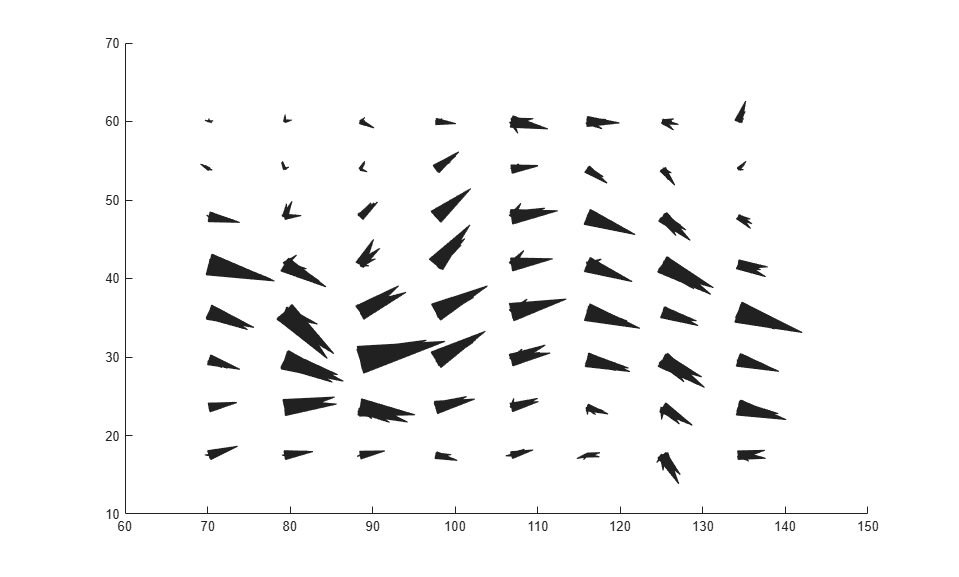```hcone.FaceColor = 'red'; hcone.EdgeColor = 'none'; ```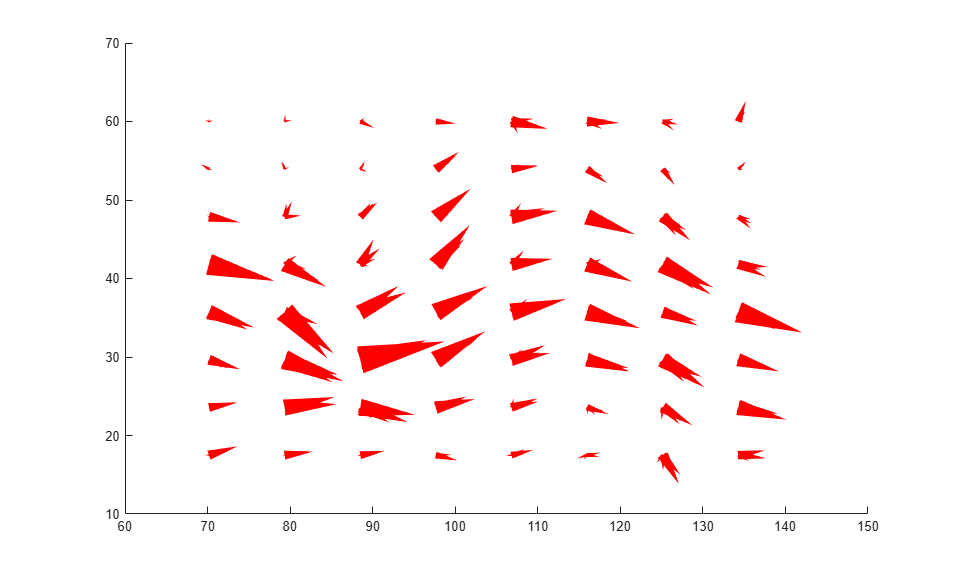```hold on wind_speed = sqrt(u.^2 + v.^2 + w.^2); ```

```hsurfaces = slice(x,y,z,wind_speed,[xmin,xmax],ymax,zmin); set(hsurfaces,'FaceColor','interp','EdgeColor','none') hold off ```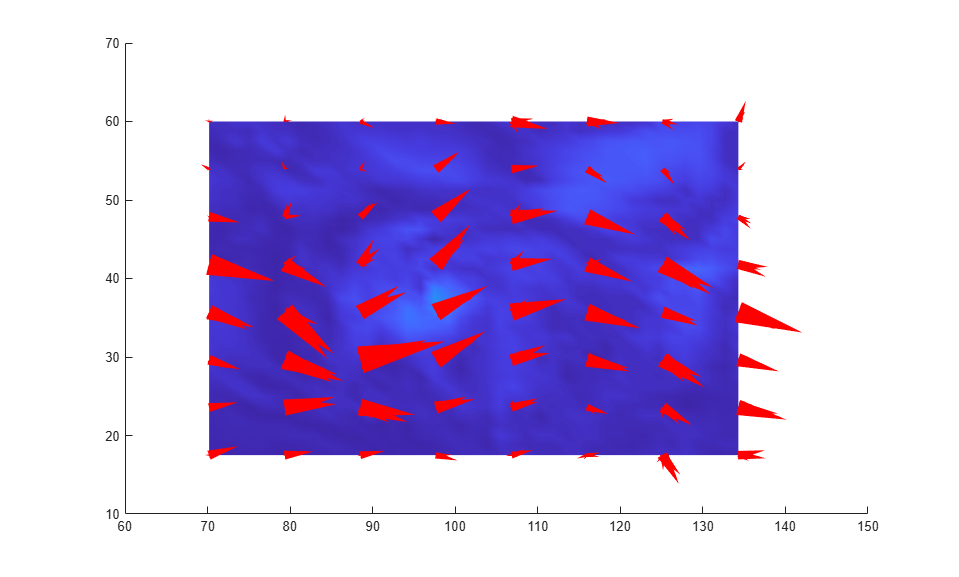```view(30,40) daspect([2,2,1]) ```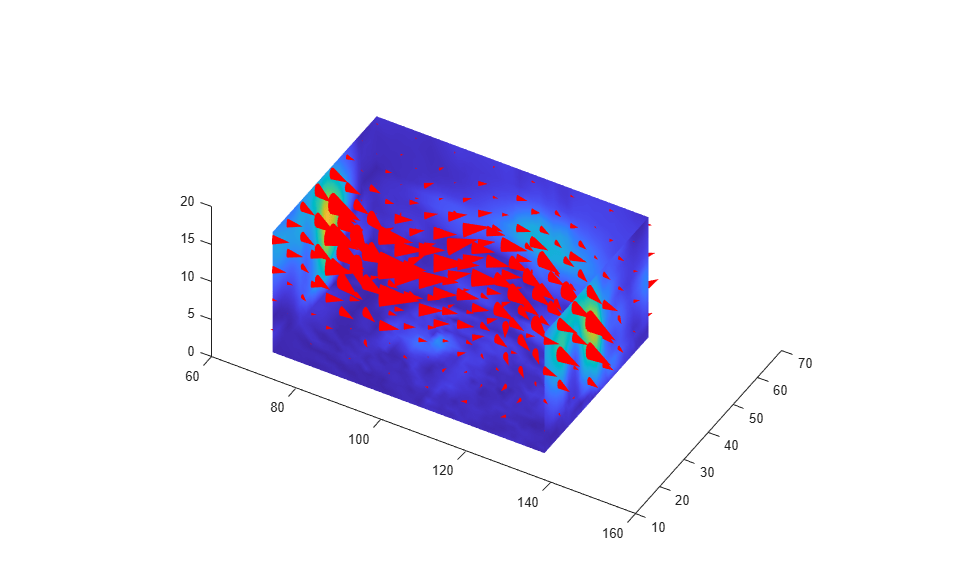```camlight right lighting gouraud set(hsurfaces,'AmbientStrength',0.6) hcone.DiffuseStrength = 0.8; ```